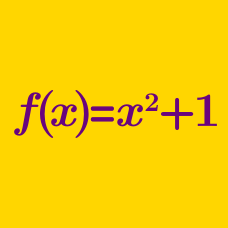Algebra

# Function Terminology

If we have a real-valued function $f(x) = x^2 + 27$, the values of $f(x)$ will necessarily fall in the following range:

$a \leq f(x).$

What is the value of $a$?

Consider two sets: $X = \{ a, b, c, d \}, \ Y = \{ 1, 2, 3 \}.$

If a function is defined from $X$ to $Y$, what is the maximum possible number of such functions?

For two sets $X=\{a,b,c\}, Y=\{7, 11, 13, 17, 20, 32\},$ $f$ is an injective function from $X$ to $Y$. If $f(a)=7$ and $f(b)=17$, what is the sum of all the elements of $Y$ that can possibly be the value of $f(c)$?

Given that the domain and codomain of the function $y=\sqrt{169-x^2}$ are restricted to the real numbers, how many elements of the domain of $y=\sqrt{169-x^2}$ are integers?

For two sets $X=\{-1,1,a\}, Y=\{3,4, b\},$ $f(x)=x^3+4$ is a bijective function from $X$ to $Y$. What is the value of $a+b$?

×

Problem Loading...

Note Loading...

Set Loading...